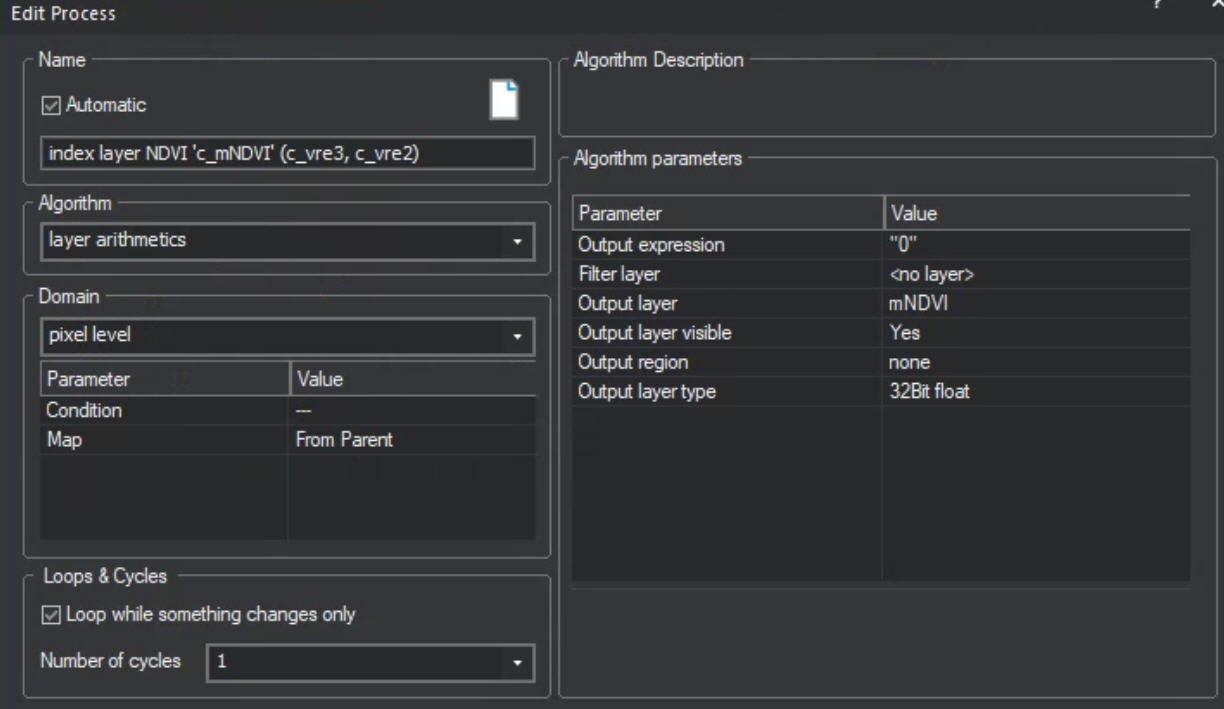I want to create a complex equation (like raster calculator in Arc). According to the YOuTube video on layer arithmetic and the help manual, there should be an "Output Value" line where I put in the equation? If not that line, where would I put the equation? I have version 10.2.0.1 comment

•Keith Peterson

The algorithm was reworked to make it easier to understand, unfortunately the video material is not up-to-date yet. You need to enter the equation in the "Output expression" field now.